Next: 3.3.2 Simulations Up: 3.3 Extension of the Previous: 3.3 Extension of the

## 3.3.1 Algorithm

The mixture of probabilistic PCA is also composed of m local PCA units, which have a center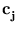, eigenvectors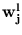, eigenvalues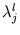, and a residual variance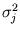. In the beginning, the centers are initialized with a vector quantizer. Then, the likelihood is maximized using an expectation-maximization iteration (section 2.3.1). As it is common to all Gaussian mixture models without annealing, this iteration may get stuck in a local optimum. Therefore, a proper initialization of the centers is important. However, this initialization is not mentioned in Tipping and Bishop (1999). Thus, the first important modification is to use Neural Gas to obtain the initial position of the centers. For Neural Gas, the standard parameter set was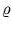(0) = 0.1m,(tmax) = 0.0001,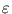(0) = 0.5,(tmax) = 0.05, and tmax = 3000 m (for all of this thesis except of section 3.3.2).

The algorithm iterates an expectation and a maximization step. In the expectation step, the posterior probabilities are computed (section 2.3.1). In the maximization step, the eigenvectors, the eigenvalues, and the residual variance of the units are computed. Here, different from Tipping and Bishop (1999), RRLSA as described in section 3.2.1 is used to compute the eigenvectors and eigenvalues. For all experiments in this thesis except the tests in section 3.3.2, in total, 40 expectation and 40 maximization steps were iterated.

During each maximization step, the algorithm goes through a fixed number of RRLSA steps (30 times the number of training patterns). For each of these steps, a pattern is drawn from the set {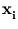}. Then, given the pattern, a unit j is randomly chosen depending on its posterior probability P(j|). For this unit the eigenvectors and eigenvalues are updated using (3.6), (3.7), and (3.8), as before.

The learning rate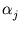in RRLSA is computed for each unit separately. Let tj be the number of times the unit j was chosen. At the beginning of each maximization step, tj restarts from zero. With increasing tj, the learning rate decays as= 1/tj. This decay rule guarantees that the result for each unit converges to an average over the presented patterns (appendix A.3).

After the eigenvectors and eigenvalues are computed, also the residual varianceis obtained recursively. Here, the update equations are also the same as the ones used in NGPCA, namely (3.3) and (3.4). The learning rateis computed as above. The principal components were extracted before computingbecause (3.3) relies on orthonormal vectors. At the beginning of the algorithm, the entries of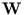were set to random values, and then,was made orthonormal. The eigenvalues and the residual variance were initially set to one.

Using an on-line algorithm like RRLSA is of advantage since for each randomly drawn pattern, random noise can be added. If a unit has in its neighborhood only a few patterns the variance in some directions will be zero. Thus, the PCA yields eigenvalues with zero value. Computationally, such eigenvalues are a problem because the corresponding probability density has an infinite peak. With the addition of noise, however, zero variance can be avoided. In the original algorithm suggested by Tipping and Bishop (1999), the computation of the eigenvectors and eigenvalues is done by one step in batch mode. Here, the variance of single points cannot be increased by adding noise. For all experiments, the added noise for each dimension was in the interval [- 0.005;0.005].

The last modification is a correction for empty' units, these are units with a prior P(j) < 1/n. Such empty units can either result from the Neural Gas initialization (Daszykowski et al., 2002), or they can occur during the expectation-maximization iteration. In the correction, the center of the empty unit is moved near to the center of the unit with the largest prior (within a random deviation from the interval [-0.01;0.01] in the direction of the principal component). The eigenvectors, the eigenvalues, and the residual variance of the empty unit are set equal to the ones of the largest unit. Then, both units re-adjust their prior and posterior probabilities to one half the values of the former largest unit. Thus, the ellipsoids of both units overlap. The slight deviation of the centers will eventually lead to the separation of the ellipsoids in further steps of the expectation-maximization iteration (section 3.3.2). In the following, the original mixture of probabilistic PCA will be called MPPCA', and the presented extension `MPPCA-ext'.Next: 3.3.2 Simulations Up: 3.3 Extension of the Previous: 3.3 Extension of the
Heiko Hoffmann
2005-03-22